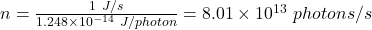## How many x-ray photons per second are created by an x-ray tube that produces a flux of x rays having a power of 1.00 W

Question

How many x-ray photons per second are created by an x-ray tube that produces a flux of x rays having a power of 1.00 W

in progress 0
2 months 2021-07-28T00:59:28+00:00 1 Answers 5 views 0

1. Complete question:

How many x-ray photons per second are created by an x-ray tube that produces a flux of x rays having a power of 1.00 W. Assume the average energy per photon in 78.0 keV.

The number of x-ray photons per second created by the x-ray tube is 8.01 x 10¹³ photons/sec

Explanation:

Given;

power of the flux produced, P = 1 W = 1 J/s

energy per photon, E = 78 keV

Convert the energy per photon to J

E = 78 x 10³ x 1.6 x 10⁻¹⁹ = 1.248 x 10⁻¹⁴ J / photon

let the number of photons = n

n(1.248 x 10⁻¹⁴ J / photon) = 1 J/sTherefore, the number of x-ray photons per second created by the x-ray tube is 8.01 x 10¹³ photons/sec